# Binary tree, Definition and its properties

Learn: In this article we are going to study about the basics of binary tree. We study different types of binary tree like complete Binary Tree, Strictly Binary Tree, Extended Binary Tree, And Full Binary Tree? What are the uses of binary tree? How binary tree is different from general tree?
Submitted by Amit Shukla, on October 06, 2017

I would like to request you to please visit Introduction to trees and its terminologies. This will give you the idea of tree terminologies which are we going to use in this article.

## Binary Tree

A binary tree is a finite set of nodes that is either empty or consist a root node and two disjoint binary trees called the left subtree and the right subtree.

In other words, a binary tree is a non-linear data structure in which each node has maximum of two child nodes. The tree connections can be called as branches.

According to graph theory binary trees defined here are actually arborescence. A binary tree is also known as old programming term bifurcating arborescence, before the modern computer science terminology prevailed.Binary tree is also known as rooted binary tree because some author uses this term to emphasize the fact that the tree is rooted, but as defined above, a binary tree is always rooted. A binary tree is a special case of an ordered binary tree, where k is 2.

1. Trees are used to represent data in hierarchical form.
2. Binary tree is the one in which each node has maximum of two child- node.
3. The order of binary tree is ‘2’.
4. Binary tree does not allow duplicate values.
5. While constructing a binary, if an element is less than the value of its parent node, it is placed on the left side of it otherwise right side.
6. A binary tree is shown for the element 40, 56, 35, 48, 22, 65, 28.

Following there is an example of binary search tree: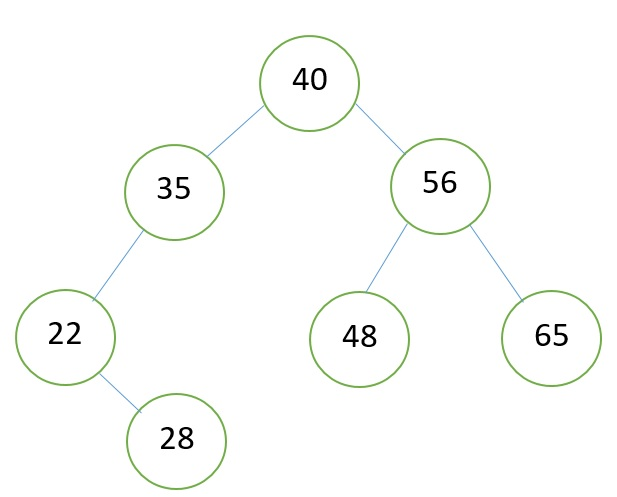1. Searching in Binary tree become faster.
2. Binary tree provides six traversals.
3. Two of six traversals give sorted order of elements.
4. Maximum and minimum elements can be directly picked up.
5. It is used for graph traversal and to convert an expression to postfix and prefix forms.

1) Complete Binary Tree

A binary tree T is said to be complete binary tree if -

1. All its levels, except possibly except possibly the last, have the maximum number of nodes and
2. All the nodes at the last level appears as far left as possible.

Consider the following tree, which is complete binary tree: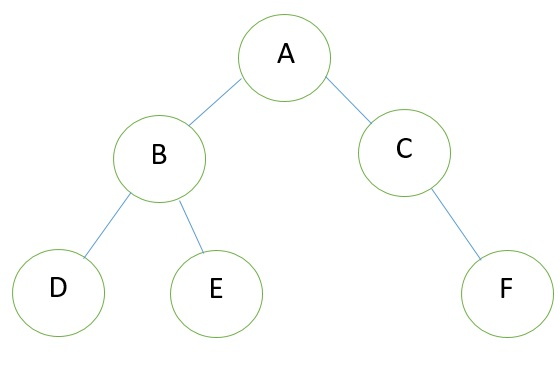Note: Full binary tree is also called complete binary tree.

IF L is the level of complete binary tree then 2L – 1 nodes present in the tree.

2) Strictly Binary Tree

When every non leaf node in a binary tree is filled with left and right subtrees, the tree is called a strictly binary tree.

Following figure shows a strictly binary tree: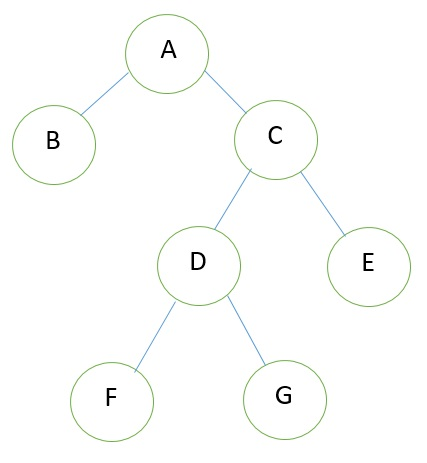In above figure nodes A, C and D provide two nodes each.

3) Extended Binary Tree

The binary tree that is extended with zero (no nodes) or left or right node or both the nodes is called an extended binary tree or a 2- tree.

The extended binary tree is shown in figure above. The extended nodes are indicated by square box. Maximum nodes in the binary tree have one child (nodes) shown at either left or right by adding one or more children, the tree can be extended. The extended binary tree is very useful for representation of algebraic expressions. The left and right child nodes denote operands and parent node indicates operator.

Example of Tree without Extension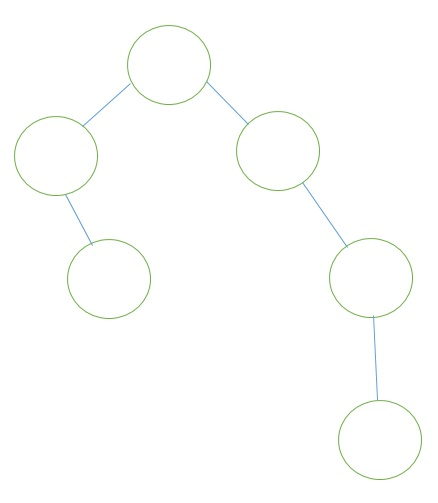Example of Tree with Extension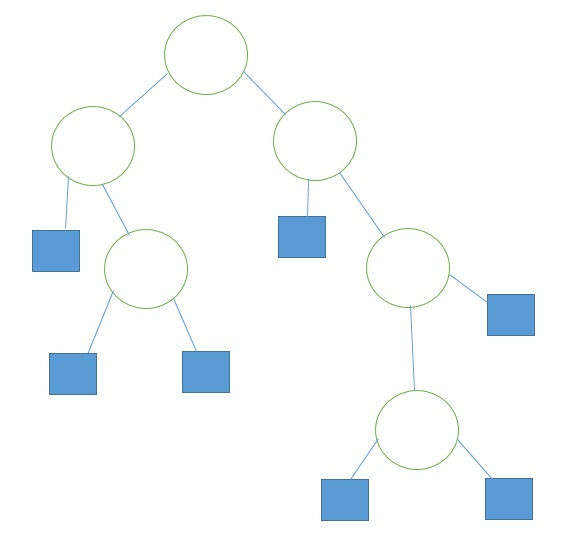4) Full Binary Tree

A Binary Tree is full binary tree if and only if -

1. Each non- leaf node has exactly two child nodes.
2. All leaf nodes are at the same level.

For Example - Consider the following tree, which is full binary tree of height 2.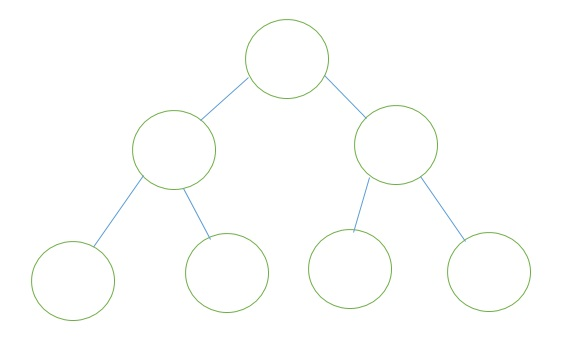Properties of Full Binary Tree

1. A binary tree of height h with no missing node.
2. All leaves are at level h and all other nodes have two children.
3. All the nodes that are at a level less than h have two children each.